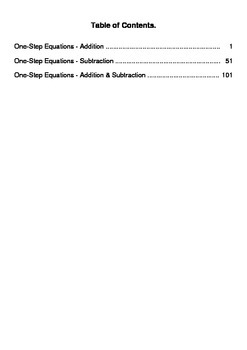digital

# One Step Equations - Addition & Subtraction WorksheetsSubject
Resource Type
Formats

TpT Digital Activity

PDF (10 MB|302 pages)
\$4.00
TpT Digital Activity
Add notes & annotations through an interactive layer and assign to students via Google Classroom.
\$4.00
TpT Digital Activity
Add notes & annotations through an interactive layer and assign to students via Google Classroom.

### Description

One Step Equations - Addition & Subtraction Worksheets.
Package includes 150 worksheets.

One-Step Equations - Addition (50 Worksheets)
Example:
x + 5 = 10

One-Step Equations - Subtraction (50 Worksheets)
Example:
x - 2 = 4

One-Step Equations - Addition & Subtraction (50 Worksheets)
Example:
x + 2 = 7
x - 1 = 9

You can purchase all One-Step Equations Operations Worksheets (Addition, Subtraction, Multiplication and Division Worksheets) and save your money! One-Step Equations 6\$

----------------------------------

You May Also Like These Worksheets:
One-Step Equations - All Basic Operations
One-Step Equations - Addition & Subtraction
One-Step Equations - Multiplication & Division
Two-Step Equations

You May Also Like These Task Cards:

Be the first to know about my new discounts, freebies and product launches:
Total Pages
302 pages
Included
Teaching Duration
N/A
Report this Resource to TpT
Reported resources will be reviewed by our team. Report this resource to let us know if this resource violates TpT’s content guidelines.